Open in App
Not now

# How to Drop Rows with NaN Values in Pandas DataFrame?

• Difficulty Level : Easy
• Last Updated : 01 Sep, 2021

NaN stands for Not A Number and is one of the common ways to represent the missing value in the data. It is a special floating-point value and cannot be converted to any other type than float. NaN value is one of the major problems in Data Analysis. It is very essential to deal with NaN in order to get the desired results. In this article, we will discuss how to drop rows with NaN values.
We can drop Rows having NaN Values in Pandas DataFrame by using dropna() function

` df.dropna() `

It is also possible to drop rows with NaN values with regard to particular columns using the following statement:

`df.dropna(subset, inplace=True)`

With in place set to True and subset set to a list of column names to drop all rows with NaN under those columns.

Example 1:

## Python3

 `# importing libraries``import` `pandas as pd``import` `numpy as np` `num ``=` `{``'Integers'``: [``10``, ``15``, ``30``, ``40``, ``55``, np.nan,``                    ``75``, np.nan, ``90``, ``150``, np.nan]}` `# Create the dataframe``df ``=` `pd.DataFrame(num, columns ``=``[``'Integers'``])` `# dropping the rows having NaN values``df ``=` `df.dropna()` `# printing the result``df`

Output: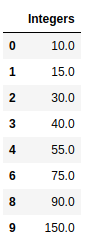Note: We can also reset the indices using the method reset_index()

`df = df.reset_index(drop=True)`

Example 2:

## Python3

 `# importing libraries``import` `pandas as pd``import` `numpy as np` `nums ``=` `{``'Integers_1'``: [``10``, ``15``, ``30``, ``40``, ``55``, np.nan,``                       ``75``, np.nan, ``90``, ``150``, np.nan],``           ``'Integers_2'``: [np.nan, ``21``, ``22``, ``23``, np.nan,``                          ``24``, ``25``, np.nan, ``26``, np.nan,``                          ``np.nan]}` `# Create the dataframe``df ``=` `pd.DataFrame(nums, columns ``=``[``'Integers_1'``, ``'Integers_2'``])` `# dropping the rows having NaN values``df ``=` `df.dropna()` `# To reset the indices``df ``=` `df.reset_index(drop ``=` `True``)` `# Print the dataframe``df`

Output: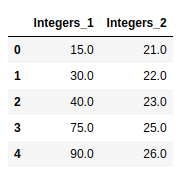Example 3:

## Python3

 `# importing libraries``import` `pandas as pd``import` `numpy as np` `nums ``=` `{``'Student Number'``: [ ``1001``, ``1111``, ``1202``, ``1229``, ``1330``,``                           ``1335``, np.nan, ``1400``, ``1150``, np.nan],``           ``'Seat Number'``: [np.nan, ``15``, ``22``, ``43``, np.nan, ``44``,``                           ``55``, np.nan, ``57``, np.nan]}` `# Create the dataframe``df ``=` `pd.DataFrame(nums, columns ``=``[``'Student Number'``, ``'Seat Number'``])` `# dropping the rows having NaN values``df ``=` `df.dropna()` `# To reset the indices``df ``=` `df.reset_index(drop ``=` `True``)` `# Print the dataframe``df`

Output: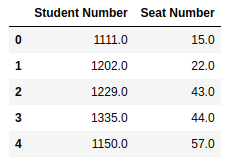Example 4:

## Python3

 `# importing libraries``import` `pandas as pd``import` `numpy as np` `car ``=` `{``'Year of Launch'``: [ ``1999``, np.nan, ``1986``, ``2020``, np.nan,``                          ``1991``, ``2007``, ``2011``, ``2001``, ``2017``],``           ``'Engine Number'``: [np.nan, ``15``, ``22``, ``43``, ``44``, np.nan,``                             ``55``, np.nan, ``57``, np.nan],``        ``'Chasis Unique Id'``: [``4023``, np.nan, ``3115``, ``4522``, ``3643``,``                             ``3774``, ``2955``, np.nan, ``3587``, np.nan]}` `# Create the dataframe``df ``=` `pd.DataFrame(car, columns ``=``[``'Year of Launch'``, ``'Engine Number'``,``                                 ``'Chasis Unique Id'``])` `# dropping the rows having NaN values``df ``=` `df.dropna()` `# To reset the indices``df ``=` `df.reset_index(drop ``=` `True``)` `# Print the dataframe``df`

Output: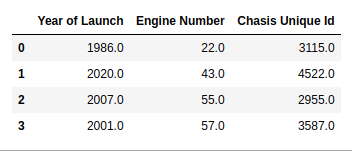Example 5:

## Python3

 `# Importing libraries``import` `pandas as pd``import` `numpy as np` `# Creating a dictionary``dit ``=` `{``'August'``: [``10``, np.nan, ``34``, ``4.85``, ``71.2``, ``1.1``],``       ``'September'``: [np.nan, ``54``, ``68``, ``9.25``, pd.NaT, ``0.9``],``       ``'October'``: [np.nan, ``5.8``, ``8.52``, np.nan, ``1.6``, ``11``],``       ``'November'``: [pd.NaT, ``5.8``, ``50``, ``8.9``, ``77``, pd.NaT]}` `# Converting it to data frame``df ``=` `pd.DataFrame(data``=``dit)` `# Dropping the rows having NaN/NaT values``# when threshold of nan values is 2``df ``=` `df.dropna(thresh``=``2``)` `# Resetting the indices using df.reset_index()``df ``=` `df.reset_index(drop``=``True``)` `df`

Output: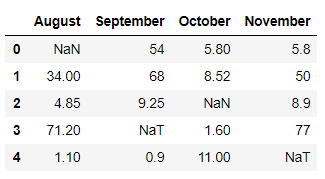In the above example, we used thresh = 2 inside the df.dropna() function which means it will drop all those rows where Nan/NaT values are 2 or more than 2, others will remain as it is.

Example 6:

## Python3

 `# Importing libraries``import` `pandas as pd``import` `numpy as np` `# Creating a dictionary``dit ``=` `{``'August'``: [``10``, np.nan, ``34``, ``4.85``, ``71.2``, ``1.1``],``       ``'September'``: [np.nan, ``54``, ``68``, ``9.25``, pd.NaT, ``0.9``],``       ``'October'``: [np.nan, ``5.8``, ``8.52``, np.nan, ``1.6``, ``11``],``       ``'November'``: [pd.NaT, ``5.8``, ``50``, ``8.9``, ``77``, pd.NaT]}` `# Converting it to data frame``df ``=` `pd.DataFrame(data``=``dit)` `# Dropping the rowns having NaN/NaT values``# under certain label``df ``=` `df.dropna(subset``=``[``'October'``])` `# Resetting the indices using df.reset_index()``df ``=` `df.reset_index(drop``=``True``)` `df`

Output: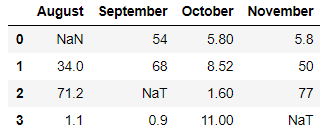In the above example, we use subset = [‘October’] inside the df.dropna() function which means it will remove all rows having Nan/NaT values under the label ‘October’.

My Personal Notes arrow_drop_up